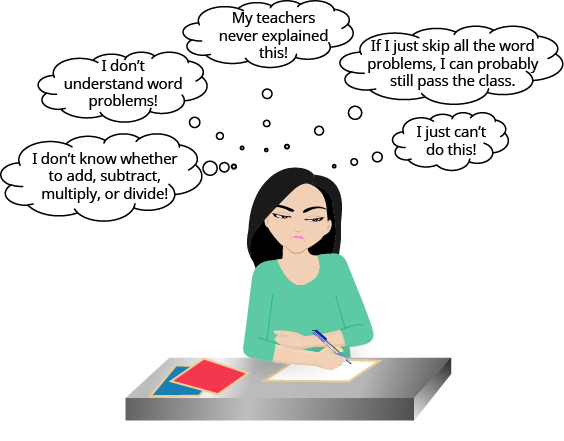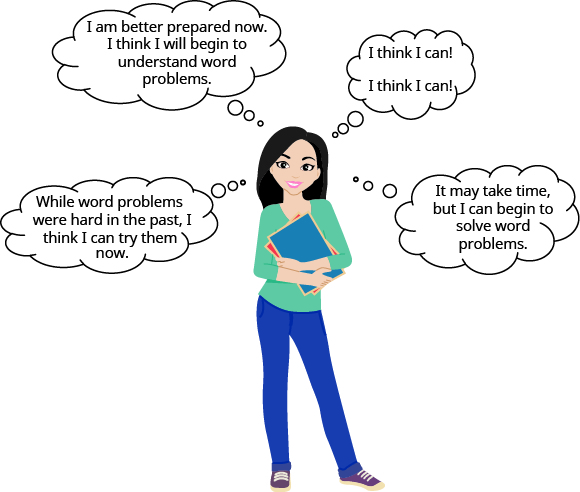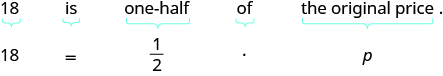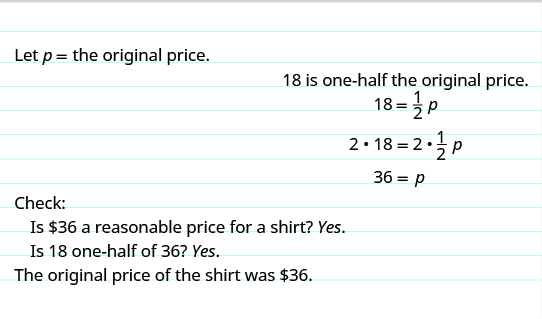## Apply a Problem-Solving Strategy to Word Problems

### Learning Outcomes

• Approach word problems with a positive attitude
• Use a problem solving strategy for word problems
• Translate more complex word problems into algebraic expressions and equations

### Approach Word Problems with a Positive Attitude

The world is full of word problems. How much money do I need to fill the car with gas? How much should I tip the server at a restaurant? How many socks should I pack for vacation? How big a turkey do I need to buy for Thanksgiving dinner, and what time do I need to put it in the oven? If my sister and I buy our mother a present, how much will each of us pay?

Now that we can solve equations, we are ready to apply our new skills to word problems. Do you know anyone who has had negative experiences in the past with word problems? Have you ever had thoughts like the student in the cartoon below?Negative thoughts about word problems can be barriers to success.

When we feel we have no control, and continue repeating negative thoughts, we set up barriers to success. We need to calm our fears and change our negative feelings.

Start with a fresh slate and begin to think positive thoughts, like the student in the cartoon below. Read the positive thoughts and say them out loud.When it comes to word problems, a positive attitude is a big step toward success.

If we take control and believe we can be successful, we will be able to master word problems.

Think of something that you can do now but couldn’t do three years ago. Whether it’s driving a car, snowboarding, cooking a gourmet meal, or speaking a new language, you have been able to learn and master a new skill. Word problems are no different. Even if you have struggled with word problems in the past, you have acquired many new math skills that will help you succeed now!

### Use a Problem-Solving Strategy for Word Problems

In earlier chapters, you translated word phrases into algebraic expressions, using some basic mathematical vocabulary and symbols. Since then, you’ve increased your math vocabulary as you learned about more algebraic procedures, and you’ve had more practice translating from words into algebra.

You have also translated word sentences into algebraic equations and solved some word problems. The word problems applied math to everyday situations. You had to restate the situation in one sentence, assign a variable, and then write an equation to solve. This method works as long as the situation is familiar to you and the math is not too complicated.

Now we’ll develop a strategy you can use to solve any word problem. This strategy will help you become successful with word problems. We’ll demonstrate the strategy as we solve the following problem.

Pete bought a shirt on sale for $$18$, which is one-half the original price. What was the original price of the shirt? Solution: Step 1. Read the problem. Make sure you understand all the words and ideas. You may need to read the problem two or more times. If there are words you don’t understand, look them up in a dictionary or on the Internet. • In this problem, do you understand what is being discussed? Do you understand every word? Step 2. Identify what you are looking for. It’s hard to find something if you are not sure what it is! Read the problem again and look for words that tell you what you are looking for! • In this problem, the words “what was the original price of the shirt” tell you what you are looking for: the original price of the shirt. Step 3. Name what you are looking for. Choose a variable to represent that quantity. You can use any letter for the variable, but it may help to choose one that helps you remember what it represents. • Let $p=$ the original price of the shirt Step 4. Translate into an equation. It may help to first restate the problem in one sentence, with all the important information. Then translate the sentence into an equation.Step 5. Solve the equation using good algebra techniques. Even if you know the answer right away, using algebra will better prepare you to solve problems that do not have obvious answers.  Write the equation. $18=\Large\frac{1}{2}p$ Multiply both sides by 2. $\color{red}{2}\cdot18=\color{red}{2}\cdot\Large\frac{1}{2}\normalsize p$ Simplify. $36=p$ Step 6. Check the answer in the problem and make sure it makes sense. • We found that $p=36$, which means the original price was $\text{\36}$. Does $\text{\36}$ make sense in the problem? Yes, because $18$ is one-half of $36$, and the shirt was on sale at half the original price. Step 7. Answer the question with a complete sentence. • The problem asked “What was the original price of the shirt?” The answer to the question is: “The original price of the shirt was $\text{\36}$.” If this were a homework exercise, our work might look like this:We list the steps we took to solve the previous example. ### Problem-Solving Strategy 1. Read the word problem. Make sure you understand all the words and ideas. You may need to read the problem two or more times. If there are words you don’t understand, look them up in a dictionary or on the internet. 2. Identify what you are looking for. Determine the constants and variables in the problem. A constant is a number in the problem that is not going to change. A variable is a number that you don’t yet know its value. 3. Name what you are looking for. Choose a letter to represent that quantity. 4. Translate words into algebraic expressions and equations. Write an equation to represent the problem. It may be helpful to first restate the problem in one sentence before translating. 5. Solve the equation using good algebra techniques. 6. Check the answer in the problem. Make sure it makes sense. 7. Answer the question with a complete sentence. ## Translate word problems into expressions One of the first steps to solving word problems is converting an English sentence into a mathematical sentence. In the table below, words or phrases commonly associated with mathematical operators are categorized. Word problems often contain these or similar words, so it’s good to see what mathematical operators are associated with them. Addition $+$ Subtraction $-$ Multiplication $\times$ Variable ? Equals $=$ More than Less than Double A number Is Together In the past Product Often, a value for which no information is given. The same as Sum Slower than times After how many hours? Total The remainder of How much will it cost? In the future Difference Faster than Some examples follow: • “$x\text{ is }5$” becomes $x=5$ • “Three more than a number” becomes $x+3$ • “Four less than a number” becomes $x-4$ • “Double the cost” becomes $2\cdot\text{ cost }$ • “Groceries and gas together for the week cost$250” means $\text{ groceries }+\text{ gas }=250$
• “The difference of $9$ and a number” becomes $9-x$. Notice how $9$ is first in the sentence and the expression.

Let’s practice translating a few more English phrases into algebraic expressions.

### Example

Translate the table into algebraic expressions:

 some number the sum of the number and $3$ twice the sum of the number and $3$ a length double the length double the length, decreased by $6$ a cost the difference of the cost and $20$ $2$ times the difference of the cost and $20$ some quantity the difference of $5$ and the quantity the difference of $5$ and the quantity, divided by $2$ an amount of time triple the amount of time triple the amount of time, increased by $5$ a distance the sum of $-4$ and the distance the sum of $-4$ and the twice the distance

In this example video, we show how to translate more words into mathematical expressions.

For another review of how to translate algebraic statements into words, watch the following video.

The power of algebra is how it can help you model real situations in order to answer questions about them.  Let’s use this approach with another example.

### Example

Yash brought apples and bananas to a picnic. The number of apples was three more than twice the number of bananas. Yash brought $11$ apples to the picnic. How many bananas did he bring?

### Example

Twenty-eight less than five times a certain number is $232$. What is the number?

In the video that follows, we show another example of how to translate a sentence into a mathematical expression using a problem solving method.

In the next example, we will apply our Problem-Solving Strategy to applications of percent.

### example

Nga’s car insurance premium increased by $\text{\60}$, which was $\text{8%}$ of the original cost. What was the original cost of the premium?

## Contribute!

Did you have an idea for improving this content? We’d love your input.